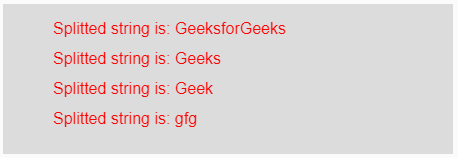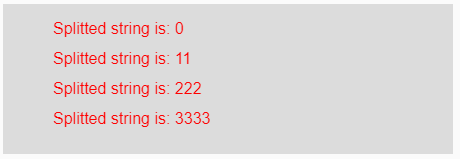# p5.js | split() function

• Last Updated : 22 Apr, 2019

The split() function in p5.js is used to break the input string into pieces using a delimiter. This delimiter could be any string or symbol used between each piece of the input string.

Syntax:

Hey geek! The constant emerging technologies in the world of web development always keeps the excitement for this subject through the roof. But before you tackle the big projects, we suggest you start by learning the basics. Kickstart your web development journey by learning JS concepts with our JavaScript Course. Now at it's lowest price ever!

`split(String, Delimiter)`

Parameters: This function accepts two parameters which are described below:

• String: This is the input string which are to be splitted.
• Delimiter: This is any string or symbol used to separate the data of the input string.

Return Value: It returns the splitted data of the input string.

Below programs illustrate the split() function in p5.js.

Example 1: This example uses split() function to break the input string into pieces of substring using delemeter.

 `function` `setup() { ``  ` `    ``// Creating Canvas size``    ``createCanvas(450, 150); ``} ``function` `draw() { ``      ` `    ``// Set the background color ``    ``background(220); ``    ` `    ``// Initializing the Strings``    ``let String = ``'GeeksforGeeks/Geeks/Geek/gfg'``;  ``     ` `    ``// Calling to split() function.``    ``let A = split(String, ``'/'``);``     ` `    ``// Set the size of text ``    ``textSize(16); ``      ` `    ``// Set the text color ``    ``fill(color(``'red'``)); ``    ` `    ``// Getting splitted string``    ``text(``"Splitted string is: "` `+ A, 50, 30);  ``    ``text(``"Splitted string is: "` `+ A, 50, 60);  ``    ``text(``"Splitted string is: "` `+ A, 50, 90);  ``    ``text(``"Splitted string is: "` `+ A, 50, 120);``} `

Output:Example 2: This example uses split() function to break the input string into pieces of substring using delemeter.

 `function` `setup() { ``  ` `    ``// Creating Canvas size``    ``createCanvas(450, 150); ``} ``function` `draw() { ``      ` `    ``// Set the background color ``    ``background(220); ``    ` `    ``// Initializing the Strings``    ``let String = ``'0&11&222&3333'``;  ``     ` `    ``// Calling to split() function.``    ``let A = split(String, ``'&'``);``     ` `    ``// Set the size of text ``    ``textSize(16); ``      ` `    ``// Set the text color ``    ``fill(color(``'red'``)); ``    ` `    ``// Getting splitted string``    ``text(``"Splitted string is: "` `+ A, 50, 30);  ``    ``text(``"Splitted string is: "` `+ A, 50, 60);  ``    ``text(``"Splitted string is: "` `+ A, 50, 90);  ``    ``text(``"Splitted string is: "` `+ A, 50, 120);``} `

Output:Reference: https://p5js.org/reference/#/p5/split

My Personal Notes arrow_drop_up